• 链表冒泡排序 比较两个相邻的元素大小，每一趟会把较大（或较小）的数放在往后移。链表冒泡排序思想：设置两个指针，一个是当前指针，一个是尾指针，当前的指针指向头节点，将尾指针赋为空，当当前的指针不等于尾...
链表冒泡排序
比较两个相邻的元素大小，每一趟会把较大（或较小）的数放在往后移。链表冒泡排序思想：设置两个指针，一个是当前指针，一个是尾指针，当前的指针指向头节点，将尾指针赋为空，当当前的指针不等于尾指针是一次循环，第一次将当前一个节点的值与下一个节点的值相比较，直到下一个节点和尾指针相等（即为空），结束本次循环，最后当前的指针赋给尾指针，当前指针重新指向头结点，再两两相比较，把最大（或最小）的数放在最后

//冒泡排序的主要思想两两相性比较，每比较一次会把一轮最大或最小的数放在最后
struct student *cur,*tail;
tail=NULL;
if(cur==NULL||cur->next==NULL){
return;
}
while(cur!=tail){
while(cur->next!=tail){
if(cur->score>cur->next->score){
int temp=cur->score;
cur->score=cur->next->score;
cur->next->score=temp;
long temp1=cur->num;
cur->num=cur->next->num;
cur->next->num=temp1;
}
cur=cur->next;
}
tail=cur;
}

}
选择排序：

选择排序的思想将当前的元素和后面的元素相比较，每循环一次把最大或最小的元素赋值给当前的元素。
//选择排序
struct student *cur,*p=NULL,*q=NULL;
int score=0,cscore=0;
if(cur==NULL||cur->next==NULL){
return;
}
while(cur!=NULL){
p=cur->next;
q=cur->next;
score=cur->score;
cscore=score;
while(p!=NULL){
if(cscore>p->score){
cscore=p->score;
q=p;
}
p=p->next;
}
if(score!=cscore){
q->score=score;
cur->score=cscore;
long temp=cur->num;
cur->num=q->num;
q->num=temp;
}
cur=cur->next;
}
} 链表排序的源代码（可测）：#include <stdio.h>
#include <malloc.h>
#define LEN sizeof(struct student)

struct student{
long num;
int score;
struct student *next;
};

int main(){
struct student *create();

}
int n;
struct student *create(){
n=0;
p1=p2=(struct student *)malloc(LEN);
scanf("%ld,%d",&p1->num,&p1->score);
while(p1->num!=0){
n=n+1;
if(n==1){
}else{
p2->next=p1;
}
p2=p1;
p1=(struct student *)malloc(LEN);
scanf("%ld,%d",&p1->num,&p1->score);
}
p2->next=NULL;
}
struct student *p;
printf("%d名学生的成绩分别是：\n",n);
do{
printf("%ld,%d\n",p->num,p->score);
p=p->next;
}while(p!=NULL);
}else{
printf("链表为空");
}
}
//冒泡排序的主要思想将前后两两相比较，每比较一次会把一轮最大或最小的数放在最后
struct student *cur,*tail;
tail=NULL;
if(cur==NULL||cur->next==NULL){
return;
}
while(cur!=tail){
while(cur->next!=tail){
if(cur->score>cur->next->score){
int temp=cur->score;
cur->score=cur->next->score;
cur->next->score=temp;
long temp1=cur->num;
cur->num=cur->next->num;
cur->next->num=temp1;
}
cur=cur->next;
}
tail=cur;
}

}
//选择排序
struct student *cur,*p=NULL,*q=NULL;
int score=0,cscore=0;
if(cur==NULL||cur->next==NULL){
return;
}
while(cur!=NULL){
p=cur->next;
q=cur->next;
score=cur->score;
cscore=score;
while(p!=NULL){
if(cscore>p->score){
cscore=p->score;
q=p;
}
p=p->next;
}
if(score!=cscore){
q->score=score;
cur->score=cscore;
long temp=cur->num;
cur->num=q->num;
q->num=temp;
}
cur=cur->next;
}
} 


展开全文• ## 数据结构--链表的排序详解

万次阅读 多人点赞 2016-11-18 22:18:26
1、前言 前面两篇博客，我已经把线性表的两种基本的...2、链表排序—最简单、直接的方式（直接采用冒泡或者选择排序，而且不是交换结点，只交换数据域）//线性表的排序,采用冒泡排序,直接遍历链表 void Listsort(Nod
1、前言
前面两篇博客，我已经把线性表的两种基本的表示形式，做了一个基本的介绍和一些对比。但是，我突然发现在链表这里我缺少一个很重要的内容，那就是对我们的链表进行排序，其实，在连接两个链表的时候，就要求我们的那两个链表是有序的。

2、链表排序—最简单、直接的方式（直接采用冒泡或者选择排序，而且不是交换结点，只交换数据域）

//线性表的排序,采用冒泡排序,直接遍历链表
int i = 0;
int j = 0;
//用于变量链表
//作为一个临时量
Node * p;
Node * p1;
//如果链表为空直接返回

for (i = 0; i < head->value - 1; i++) {
for (j = 0; j < head->value - i - 1; j++) {
//得到两个值
p = L;
p1 = L->next;
//如果前面的那个比后面的那个大，就交换它们之间的是数据域
if (p->value > p1->value) {
Elemtype temp = p->value;
p->value = p1->value;
p1->value = temp;
}
L = L->next;
}
}
}

因为排序中速度比较快的如快速排序是要求它们的数据域的地址是相连接的，它比较适合于顺序结构，而链式结构的时候，我们就只有使用只会进行前后两个比较多排序算法，比如冒泡排序等。我们这里是没有交换结点的一种排序方式，这种方式简单，明了，这样就是数组排序的时候是一样的。后面我会写通过交换结点的方式的排序。
下面我们就讨论讨论这个排序算法的时间复杂度了，因为它是使用冒泡排序的，它的时间只要消耗在那两重循环，所以时间复杂度为：O(n*n),这个效率实在是太低，下面我们对这个想(ˇˍˇ) 想～通过另外一种方式来实现链表的排序

3、另外一种链表排序方式
我们在讨论排序算法的时候，都是把数据存放在数组中进行讨论的，在顺序结构下，我们可以采取很多高效的排序算法，那么这个就是我们另外一种对链表排序的方式，先把链表的内容存放到数组中（时间为O(n)），然后，我们在对那个数组进行排序（最快为nlog(n)），最后，存放链表中（时间为O(n)）。通过计算，我们可以得到它的时间复杂为（O(nlogn)）,这个速度已经和顺序结构下差不多了，可以接受

void Listsort_1(Node* & head) {
int i = 0;
int j = 0;
//用于变量链表
//如果链表为空直接返回
Elemtype * copy = new Elemtype[head->value];
//变量链表，存放数组
for (i = 0; i < head->value; i++) {
L = L->next;
copy[i] = L->value;
}
//调用STL中的sort函数
//存放回链表中
for (i = 0; i < head->value; i++) {
L = L->next;
L->value= copy[i];
}
}

4、先我们通过采取增大数据量的方式，比较两种排序在效率如何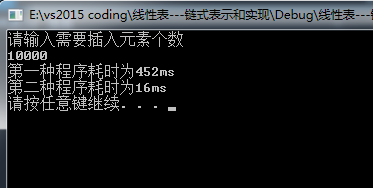如图所示，在数据量为10000的时候，明显第二种排序算法消耗的时间比第一种快了28倍左右。

5、下面通过交换结点实现链表的排序
首先我们编写交换结点的函数，结点的交换主要就是考虑结点的指针域的问题，其中相邻两个结点是一种特殊的情况，要拿出来特别考虑。我们先画出结点交换的思路图，如下图
首先我们给出相邻两个结点交换的思路：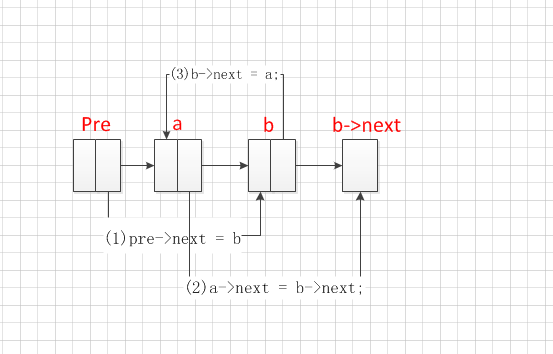下面是普通情况下的交换如下图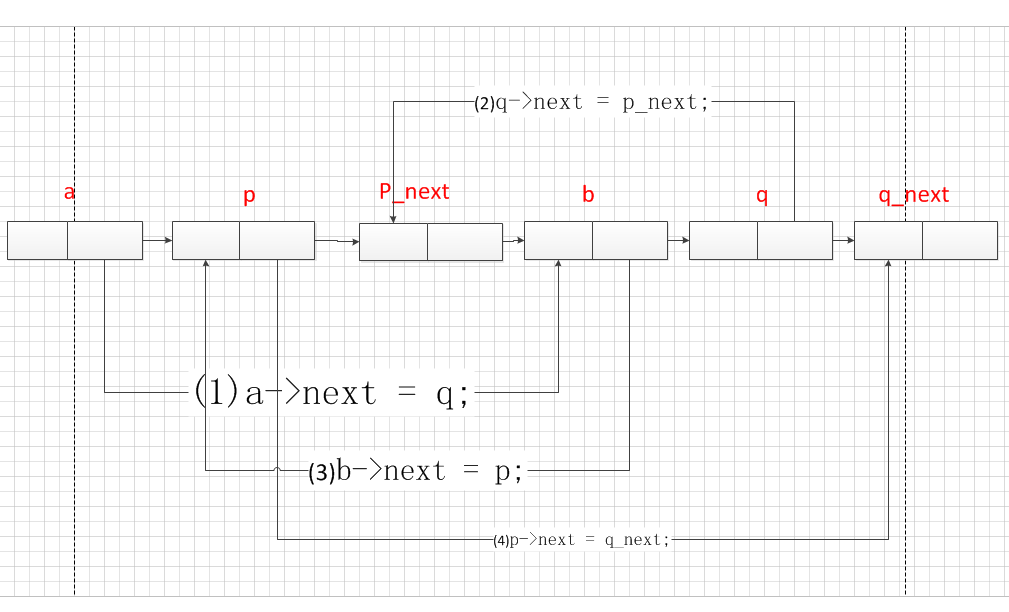//参数为头结点和需要交换的两个结点的位置（起点为1）
void swap_node(Node * & head,int i,int j) {
//位置不合法
cout << "请检查位置是否合法" << endl;
return;
}
//同一个位置不用交换
if (i == j)
{
return;
}
//相邻两个交换比较简单
if (abs(i - j) == 1) {
//位置靠前的那个结点的前一个结点
Node * pre;
if (i < j)
else

//保存第一个结点
Node * a = pre->next;
//保存第二结点
Node * b = a->next;
//改变pre下一个结点的值
pre->next = b;
//必须先把b的下一个结点值给a先
a->next = b->next;
//让b的下一个结点等于a
b->next = a;
return;
}

//第一个结点前一个结点
Node * a = getitem(head, i);
//第二个结点的前一个结点
Node * b = getitem(head, j);
//第一个结点
Node * p = a->next;
//第二个结点
Node * q = b->next;
//第一个结点的下一个结点
Node * p_next = p->next;
//第二结点的下一个结点
Node * q_next = q->next;

//a的下一个结点指向第二个结点q
a->next = q;
//第二结点的下一个结点指向第一个结点的下一个结点
q->next = p_next;
//b的下一个结点指向第一个结点p
b->next = p;
//第一个结点的下一个结点指向第二个结点的下一个结点
p->next = q_next;

}

排序时候的代码，切记交换结点都是前后结点交换，所以交换完成后，L就已经被移动到下一个结点了，故不要再执行：L=L->next

//线性表的排序，交换结点
int i = 0;
int j = 0;
//用于变量链表
//作为一个临时量
Node * p;
Node * p1;
//如果链表为空直接返回
int flag = 0;
for (i = 0; i < head->value - 1; i++) {
for (j = 0; j < head->value - 1 - i; j++) {
//如果我们交换了结点，那么我们就已经在交换结点的时候，把L移动到下一个结点了，所以不要
//再执行：L = L->next;，否则会报错的
if (L->value > L->next->value) {
flag = 1;
swap_node(head, j + 1, j + 2);

}
if (flag == 1) {
flag = 0;
}
else {
L = L->next;
}

}
}
}

好了，今天的就写到这里了，今天通过写交换结点，发现链表真的很容易忽悠人，我就被忽悠了一个小时，才知道那个结点已经被移动到下一个结点了。

最后，补充一个实现链表反转的好方法（感觉你在头文件里面计算一下链表的长度可以带来很多遍历的）

void rollback(Node * & head) {
//先知道了最后一个元素和第一个元素的位置
int start = 1;
//两边同时开工
//进行调换
while (1) {
if (end == start)
return;
--end;
++start;
}
}

希望大家，对我写的代码做出一些评价。我想想还是直接贴个完成的代码出来好了，调转代码也在里面了

#include<iostream>
#include<ctime>
#include<cstdlib>
#include<windows.h>
#include<algorithm>
using namespace std;
typedef int Elemtype;

//链式结构，我们打算在链表中添加一个
//保存长度的头结点，加入这个结点可以方便我们对结点做一些
//基本的操作，结点保存的是线性表的长度
struct Node
{
//结点的值,如果是头结点，保存是链表的长度
Elemtype value;
//下一个结点的地址
Node * next;

};

//创建一个空链表，每个头结点就代表一个链表
void InitList(Node * & head) {
}
//销毁一个链表
void DestroyList(Node * & head) {
}

//清空整个列表
void ClearList(Node * & head) {
}

//插入函数
bool Listinsert(Node * & head, int i, Elemtype value) {

//插入到前面的方法
int j = 0;
//如果插入的位置不合法，直接返回错误提示
if (i<1 || i>head->value + 1)return false;

//得到插入位置的前一个结点
while (j < i - 1) {
L = L->next;
++j;
}

//s是一个临时结点
Node * s = new Node();
s->value = value;    //先对临时结点赋值
s->next = L->next;   //让临时结点下一个位置指向当前需要插入前一个结点的下一个位置
L->next = s;          //让前一个结点下一个位置指向临时结点，完成
//线性表的长度加一
return true;
}
//得到某个位置上的值
Node * getitem(Node * & head, int i) {
//我们要求程序返回特定位置上的值
//我们一样是从头结点开始寻找该位置
int j = 0;
//想要的那个位置是否合法
if (i<1 || i >head->value)return NULL;

//同样是先得到前一个结点
while (j < i - 1) {
L = L->next;
++j;
}
//value = L->next->value;
return L;
}
//线性表的排序,采用冒泡排序,直接遍历链表
int i = 0;
int j = 0;
//用于变量链表
//作为一个临时量
Node * p;
Node * p1;
//如果链表为空直接返回

for (i = 0; i < head->value - 1; i++) {
for (j = 0; j < head->value - i - 1; j++) {
//得到两个值
p = L;
p1 = L->next;
//如果前面的那个比后面的那个大，就交换它们之间的是数据域
if (p->value > p1->value) {
Elemtype temp = p->value;
p->value = p1->value;
p1->value = temp;
}
L = L->next;
}
}
}
//通过数组来完成我的排序
int i = 0;
int j = 0;
//用于变量链表
//如果链表为空直接返回
Elemtype * copy = new Elemtype[head->value];
//变量链表，存放数组
for (i = 0; i < head->value; i++) {
L = L->next;
copy[i] = L->value;
}
//调用STL中的sort函数
//存放回链表中
for (i = 0; i < head->value; i++) {
L = L->next;
L->value = copy[i];
}
}

//参数为头结点和需要交换的两个结点的位置（起点为1）
void swap_node(Node * & head,int i,int j) {
//位置不合法
cout << "请检查位置是否合法" << endl;
return;
}
//同一个位置不用交换
if (i == j)
{
return;
}
//相邻两个交换比较简单
if (abs(i - j) == 1) {
//位置靠前的那个结点的前一个结点
Node * pre;
if (i < j)
else

//保存第一个结点
Node * a = pre->next;
//保存第二结点
Node * b = a->next;
//改变pre下一个结点的值
pre->next = b;
//必须先把b的下一个结点值给a先
a->next = b->next;
//让b的下一个结点等于a
b->next = a;
return;
}

//第一个结点前一个结点
Node * a = getitem(head, i);
//第二个结点的前一个结点
Node * b = getitem(head, j);
//第一个结点
Node * p = a->next;
//第二个结点
Node * q = b->next;
//第一个结点的下一个结点
Node * p_next = p->next;
//第二结点的下一个结点
Node * q_next = q->next;

//a的下一个结点指向第二个结点q
a->next = q;
//第二结点的下一个结点指向第一个结点的下一个结点
q->next = p_next;
//b的下一个结点指向第一个结点p
b->next = p;
//第一个结点的下一个结点指向第二个结点的下一个结点
p->next = q_next;

}
//反转
void rollback(Node * & head) {
//先知道了最后一个元素和第一个元素的位置
int start = 1;
//两边同时开工
//进行调换
while (1) {
if (end <= start)
return;
--end;
++start;
}
}
//线性表的排序,采用冒泡排序,直接遍历链表
//线性表的排序，交换结点
int i = 0;
int j = 0;
//用于变量链表
//作为一个临时量
Node * p;
Node * p1;
//如果链表为空直接返回
int flag = 0;

for (i = 0; i < head->value - 1; i++) {
for (j = 0; j < head->value - 1 - i; j++) {
//如果我们交换了结点，那么我们就已经在交换结点的时候，把L移动到下一个结点了，所以不要
//再执行：L = L->next;，否则会报错的
if (L->value > L->next->value) {
flag = 1;
swap_node(head, j + 1, j + 2);

}
if (flag == 1) {
flag = 0;
}
else {
L = L->next;
}

}
}
}

void print(Node * & head) {
//输出我们只需要传入头结点，然后循环判断当前结点下一个结点是否为空，
//这样就可以输出所有内容
while (L->next) {
L = L->next;
cout << L->value << " ";
}
cout << endl;
}
int main() {
//链表的头结点，不存放任何值，首先初始化头结点

srand((int)time(NULL));     //每次执行种子不同，生成不同的随机数
//创建一个链表

int i;
cout << "请输入需要插入元素个数" << endl;
int n;
cin >> n;//5
//cout << "请输入" << n << "个值" << endl;
for (i = 0; i < n; i++) {
Elemtype temp;
temp = rand();
if (!Listinsert(head, i + 1, temp)) {
cout << "插入元素失败" << endl;
}
if (!Listinsert(head_array, i + 1, temp)) {
cout << "插入元素失败" << endl;
}
if (!Listinsert(head_node, i + 1, temp)) {
cout << "插入元素失败" << endl;
}
if (!Listinsert(head_roll, i + 1, temp)) {
cout << "插入元素失败" << endl;
}

}
cout << "初始化结果" << endl;
cout << "反转结果" << endl;
cout << "冒泡排序（数据域交换）" << endl;
cout << "借数组为媒介进行排序（数据域交换）" << endl;
cout << "冒泡排序（结点交换）" << endl;
system("pause");
return 0;

}


运行环境：vs2015
输出结果：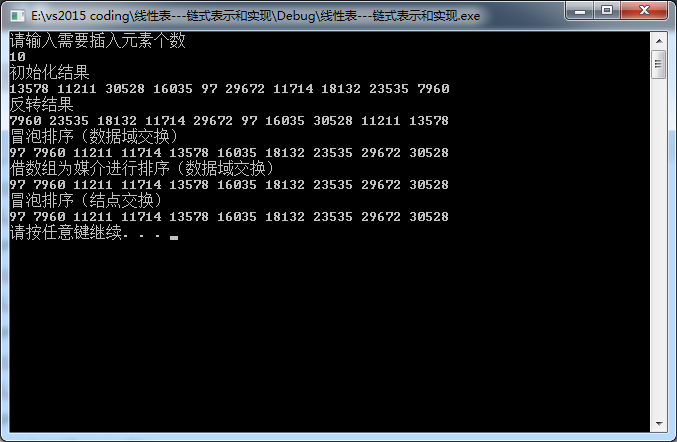展开全文数据结构
• 快速排序： 快速排序的主要思想是： 1）选定一个基准元素 2）经过一趟排序，将所有元素分成两部分 3）分别对两部分重复上述操作，直到所有元素都已排序成功 因为单链表只能从链表头节点向后遍历，没有prev指针...
快速排序： 　　 快速排序的主要思想是： 　　 1）选定一个基准元素 　　 2）经过一趟排序，将所有元素分成两部分 　　 3）分别对两部分重复上述操作，直到所有元素都已排序成功 　　 因为单链表只能从链表头节点向后遍历，没有prev指针，因此必须选择头节点作为基准元素。这样第二步操作的时间复杂度就为O(n)。由于之后都是分别对两部分完成上述操作，因此会将链表划分为lgn个段，因此时间复杂度为O(nlgn）
从中可以看出，快排实现也是先对数据进行一遍遍历找到关键值得位置，和数组不同的是数组可以从两端向中间靠拢，但是单向链表只能从一段开始，但用两个指针同样可以实现。 需要明白的一点是节点的指针就是我们在节点中定义的next指针，不要考虑的太多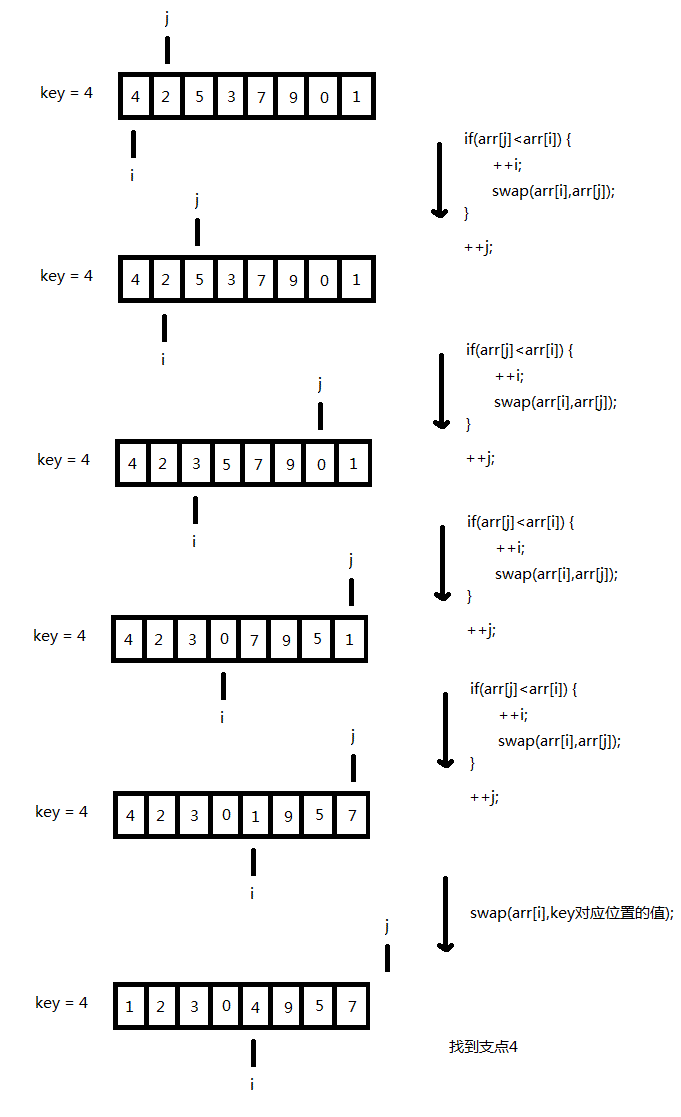//#include <iostream>
#include "stdio.h"
#include "stdlib.h"
//using namespace std;
//构造结点并初始化
typedef struct node
{
int val;
node * next;
node(int x):val(x),next(NULL){}
}mynode,*pmynode;

void swap(int* a,int * b)
{
int tmp  = *a;
* a  = *b;
* b = tmp ;
}
//定位
node *partion(node *pbegin ,node * pend)
{
if(pbegin ==pend ||pbegin->next ==pend)

return pbegin;
int mykey = pbegin ->val; //选择基准
node * p =pbegin ;
node* q =pbegin;
while(q != pend)
{
if(q->val< mykey )
{
p = p->next;
//这两种交换写法都是正确的
//swap( &(p->val) ,&(q ->val)); //小于则交换
swap( &p->val ,&q ->val); //小于则交换
}
q =q ->next;//否则一直往下走
}
swap(&p->val,&pbegin->val); //定位
return p;
}
void quick_sort(node *pbegin,node *pend)
{
if(pbegin ==pend ||pbegin->next ==pend)

return ;
node *mid =partion(pbegin,pend);
quick_sort(pbegin,mid);
quick_sort(mid->next,pend);
}

{    //如果头结点为空，则直接跳出循环
}

int main()
{
node a(1);
node b(4);
node c(6);
node d(2);
node e(5);
node f(7);
a.next = &b;
b.next = &c;
c.next = &d;
d.next = &e;
e.next = &f;

//swap( & b->val ,& c->val);
printf("%d\n", (&a)->val);
//printf("%d", &a->val);
//如果节点的指针不为空则打印节点
{

}
printf("\n");
{
}

return 0;
}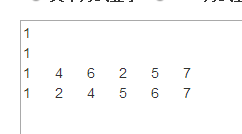展开全文• void BubbleSord(pList plist) { pNode pCur = NULL; pNode pPre = NULL; pNode pTail = NULL;//pTail的指向是这个算法的关键 if (plist == NULL || plist-&gt;next == NULL)//排除空和一个结点的情况 ...
void BubbleSord(pList plist)
{
pNode pCur = NULL;
pNode pPre = NULL;
pNode pTail = NULL;//pTail的指向是这个算法的关键
if (plist == NULL || plist->next == NULL)//排除空和一个结点的情况
{
return;
}
while (plist != pTail)//趟数
{
int IsChange = 0;//排除排序时已经是有序的，则不需要再排序
pPre = plist;
pCur = pPre->next;
while (pCur != pTail)//次数
{
if (pPre->data > pCur->data)//升序
{
int tmp = pPre->data;
pCur->data = pPre->data;
pPre->data = tmp;
IsChange = 1;
}
pPre = pPre->next;
pCur = pCur->next;
}
if (!IsChange)//如果有序了，就不排了
{
return;
}
pTail = pPre;
}
}


展开全文• ## 链表的排序

千次阅读 2019-01-07 10:32:11
链表排序 一、顺序表的排序 对顺序表的排序其实就是对结构体中的关键字的排序。 c语言版： 自定义结构体： typedef struct node { int age; int height; int width; }Node; 现在想根据其中的age...
• /********************************* 链表排序 *******************************************/  /*  ==========================   功能：选择排序(由小到大)   返回：指向链表表头的指针
• ## 链表排序算法

万次阅读 多人点赞 2018-04-18 10:34:09
排序算法概述盗个图转自：https://www.cnblogs.com/onepixel/articles/7674659.html排序算法复杂度由于是链表排序，首先定义链表节点数据结构common.htypedef struct Node LNode; struct Node { int data; LNode ...插入排序 归并排序
•   ==========================  功能：选择排序(由小到大) ... 返回：指向链表表头的指针 ========================== */ /*  选择排序的基本思想就是反复从还未排好序的那些节点中，  选出键值（就是
• #include<iostream> using namespace std; #include<string.h> typedef struct{ char name; char num; int score; }Student; typedef struct LNode{ ... LinkList Head, p, q, .
• 题目要求复杂度O(nlogn)，因此我们很自然考虑使用快速排序或者归并排序，但是后来经过实践证明，使用快速排序总是AC超时，归并排序则可以正确AC。 分析一下原因，个人认为是与测试数据有关，因为快速排序不能
• ## 一步一步写算法（之链表排序）

万次阅读 多人点赞 2011-10-25 21:09:19
相比较线性表的排序而言，链表排序的内容稍微麻烦一点。一方面，你要考虑数据插入的步骤；另外一方面你也要对指针有所顾虑。要是有一步的内容错了，那么操作系统会马上给你弹出一个exception。就链表的特殊性而言...算法 exception insert null
• ## 单链表的快速排序

万次阅读 多人点赞 2013-08-26 23:21:30
单链表的特点是：单向。...如果只知道头结点head，请问怎么将该链表排序？  设结点结构为 [cpp] view plaincopy struct Node{   int key;   Node* next;  };c++
• 现在已经给出结点定义和程序框架，包括 main 函数和链表输出函数 outlist，请编写函数 sortlist 完成链表排序功能。 函数原型 sortlist( PNODE h, int num ) 的参数含义如下：  h ：单链表的头指针 num ：新输入...c
• 1 /* 2 * FILE: sort_link.c 3 * DATE: 20180306 ... 5 * DESCRIPTION: 链表插入排序 6 */ 7 8 #include &lt;stdio.h&gt; 9 #include &lt;stdlib.h&gt; 10 11 struct node{ 12...
• 已有a、b两个链表，每个链表中的结点包括学号、成绩。要求把两个链表合并，按学号升序排列。 输入 第一行，a、b两个链表元素的数量N、M,用空格隔开。 接下来N行是a的数据 然后M行是b的数据 每行数据由学号和成绩两...
• 链表插入排序/* ========================== 功能：直接插入排序(由小到大) 返回：指向链表表 头的指针 ========================== */ /* 直接插入排序的基本思想就是假设链表的前面n-1个节点是已经按...
• #include&lt;stdio.h&gt; #include&lt;malloc.h&gt; #define LEN sizeof(struct Student) struct Student //结构体声明 { long num; int score; struct Student* next;...struct Studen...
• #include #include #include //用到了time函数 #define arraySize 10 typedef int elemType; typedef struct List { elemType elem; struct List *next;...void createRandomArray(int arr数据结构 算法 选择排序
• ## C语言—链表的排序

千次阅读 2017-01-21 12:20:11
许多人认为链表排序是这里面最难得，因为大家脑海里面对于前面插入，删除的 方法已经熟悉了，可能最容易想到的就是从结点和结点之间的联系入手，确实最 常用的就是把链表的“链”打断再重新排，再有就是重新创建一个...
• /******************************************************************** * File Name : quick_sort.c * * Created : 2007/05/08语言 c pivot null
• C语言-链表排序 题目描述 已有a、b两个链表，每个链表中的结点包括学号、成绩。要求把两个链表合并，按学号升序排列。 输入 第一行，a、b两个链表元素的数量N、M,用空格隔开。 接下来N行是a的数据 然后M行是b的...
• 链表排序有三种情况： 1、链表为空时：不用排序； 2、链表中有一个节点：不用排序； 3、链表中两个及其以上节点时：排序。 结构体节点： typedef struct student { int num; //学号 int score; //分数 ...
• ## C语言链表插入排序

千次阅读 2015-05-10 22:56:58
C语言链表插入排序 大家好，我就是人见人爱 花见花开车见爆胎的小智 声音依旧是那么低沉切性感，现在又来给大家更新博客的第一视角了。 这期给大家介绍的是链表的插入排序。 具体代码如下： struct Student *...指针
• #include&lt;stdio.h&gt; #include&lt;malloc.h&gt;//为动态分配提供函数库 typedef struct node { int num;...//创建链表 void sort();//排序 void print();//输出 node *head = ...数据结构
• 如题，复习期末时敲的，感觉敲的有点复杂 /* typedef struct List{ int i; struct List* next;...next==NULL) //如果链表为空或只有一个节点，直接返回 return head; if(head-&gt;next-&gt;...
• C语言结构体链表排序方法汇总 ========================== 功能：选择排序(由小到大) 返回：指向链表表头的指针 ========================== */ /* 选择排序的基本思想就是反复从还未排好序的那些节点中， ...
• 目录链表头结点意义链表初始化冒泡排序 链表头结点意义 头结点无实际数据，非必需，主要是为了操作的统一与方便而设立的 有头结点后，在第一个数据节点前插入、删除、修改的操作与对其它结点的操作统一了 基于带头...